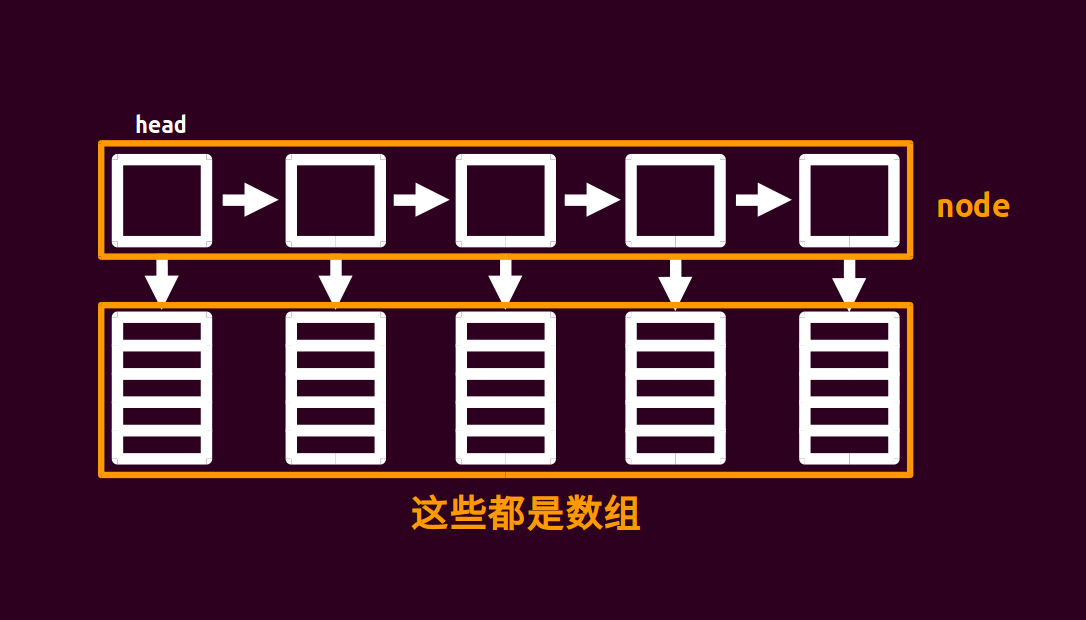# 块状链表struct node
{
node*nxt;int size;char d[(sqn<<1)+5];
node(){size=0,nxt=NULL,memset(d,0,sizeof(d));}
void pb(char c){d[size++]=c;}
};


list<vector<char> > orz_list;


# 例题

Big String POJ – 2887

#include <cstdio>
#include <cstring>
#include <cctype>
using namespace std;
static const int sqn=1e3;
struct node
{
node*nxt;int size;char d[(sqn<<1)+5];
node(){size=0,nxt=NULL;}
void pb(char c){d[size++]=c;}
char inits[(int)1e6+5];
int llen,q;
void check(node*p)
{
if(p->size>=(sqn<<1))
{
node*q=new node;
for(int i=sqn;i<p->size;i++)q->pb(p->d[i]);
p->size=sqn,q->nxt=p->nxt,p->nxt=q;
}
}
void insert(char c,int pos)
{
if(pos>llen++){while(p->nxt!=NULL)p=p->nxt;p->pb(c),check(p);return;}
tot-=p->size,cnt=pos-tot-1;
for(int i=p->size-1;i>=cnt;i--)p->d[i+1]=p->d[i];
p->d[cnt]=c,p->size++;
check(p);
}
char query(int pos)
{
node*p;int tot,cnt;
tot-=p->size;return p->d[pos-tot-1];
}
int main()
{
scanf("%s %d",inits,&q),llen=strlen(inits);
for(int i=0;i<llen;i++)
{
if(i%sqn==0&&i)p->nxt=new node,p=p->nxt;
p->pb(inits[i]);
}
char a;int k;
while(q--)
{